# Learning to Balance: Bayesian Meta-Learning for Imbalanced and Out-of-distribution Tasks

## Summary

• Propose Task-Adaptive Meta-Learning (Bayesian TAML) to solve imbalance of data or distribution with task and class, and Out-Of-Distribution Task in test data.

### Motivation

• Classic few-shot classification assume that the number of instances per task and class is fixed, which is an artificial scenario.
• In real world,
1. task imbalance : tasks that arrive at the model may have different training instances
2. class imbalance : the number of training instances per class may largely vary
3. out-of-distribution task : the new task may come from a distribution that is different from the task distribution the model has been trained on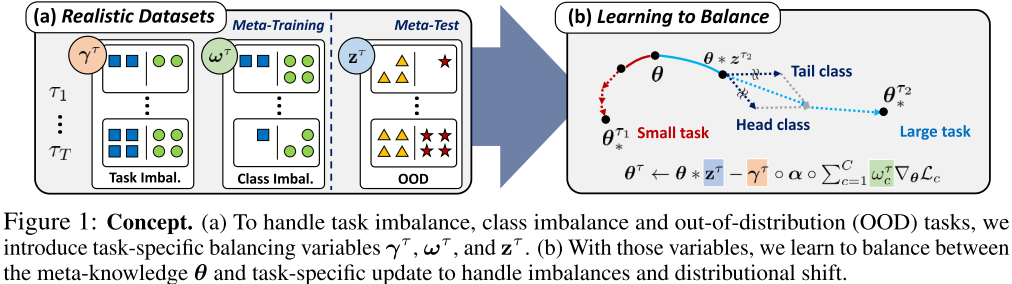### Proposal

• Tasks with small number of training data, or close to the tasks trained in meta-training step may want to rely mostly on meta-knowledge obtained over other tasks, whereas tasks that are out-of-distribution or come with more number of training data may obtain better solutions when trained in a task-specific manner.
• Propose the model to task- and class-adaptively decide how much to use from the meta-learner, and how much to learn specifically for each task and class.
• Three balancing variable:
1. class-dependent learning rate ωτ
2. task-dependent learning rate multiplier γτ
3. task-dependent modulator for initial model parameter zτ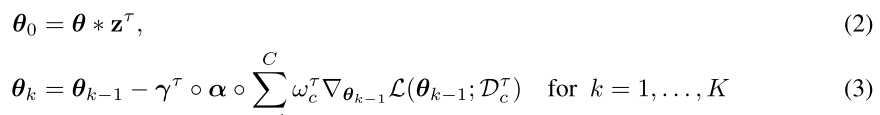• Introduce the variational inference framework for the input of the three balancing variables.
• Bayesian modeling: to maximize the conditional log-likelihood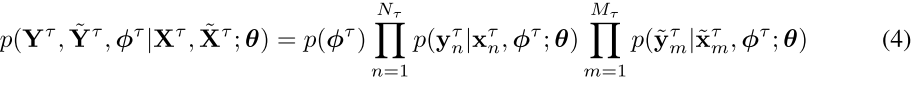#### VARIATIONAL INFERENCE

• Solving Equation (4) is intractatble, Thus, resort to amortized variational inference with a tractable form of approximate posterior.
• The final form of the meta-training minimization objective with Monte-Carlo (MC) approximation: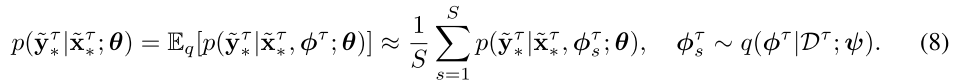• Naively approximate by taking the expectation inside for computational efficiency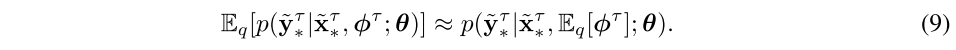### Results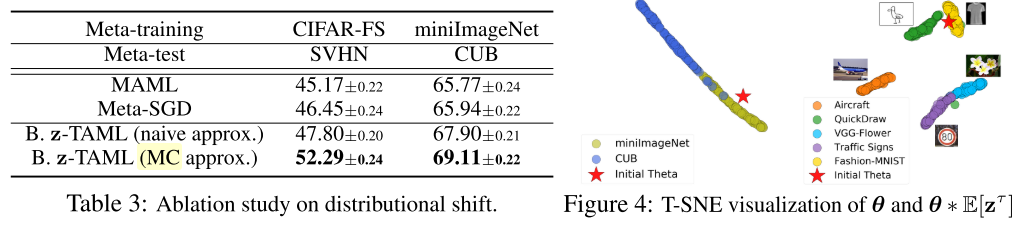• Table 3 show that the MC approximation in Eq. (8) largely outperforms the naive approximation in Eq. (9), which suggests that zτ learns very large variance.
• In Figure 4, zτ actually relocates the initial θ far from the initial parameter for these OOD tasks given at meta-test time, with larger displacements for highly heterogeneous tasks (Figure 4, right). - - This allows the model to either stick to, or deviate from the meta-knowledge based on the similarity between the tasks given at the meta-training and meta-test time.
• Try to solve the similar problem of OOD in few-shot classification problem using meta-learning :
• Modulation Network for Meta Learning
• Task-dependent modulation of batch normalization parameters
• Authors : Hae Beom Lee, Hayeon Lee, Donghyun Na, Saehoon Kim, Minseop Park, Eunho Yang, Sung Ju Hwang
• Affiliations : Kaist, Tmax
• Published : ICLR 2020 oral, Arxiv
• Code : git

## Discussion

• In seq-to-seq problem, what could be the instance of inbalance?
• Among many modulation method within meta-learning, which one would be effective to solve the discrepancy in sequence length difference between tasks?